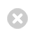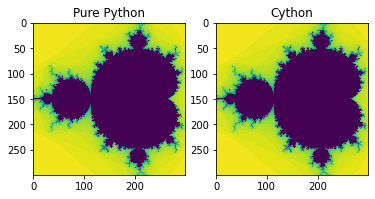Close

MPHY0021: Research Software Engineering With Python

Home## Cython¶

Cython can be viewed as an extension of Python where variables and functions are annotated with extra information, in particular types. The resulting Cython source code will be compiled into optimized C or C++ code, and thereby yielding substantial speed-up of slow Python code. In other words, Cython provides a way of writing Python with comparable performance to that of C/C++.

### Start Coding in Cython¶

Cython code must, unlike Python, be compiled. This happens in the following stages:

• The cython code in .pyx file will be translated to a C file.
• The C file will be compiled by a C compiler into a shared library, which will be directly loaded into Python.

In a Jupyter notebook, everything is a lot easier. One needs only to load the Cython extension (%load_ext Cython) at the beginning and put %%cython mark in front of cells of Cython code. Cells with Cython mark will be treated as a .pyx code and consequently, compiled into C.

For details, please see Building Cython Code.

Pure python Mandelbrot set:

In :
xmin = -1.5
ymin = -1.0
xmax = 0.5
ymax = 1.0
resolution = 300
xstep = (xmax - xmin) / resolution
ystep = (ymax - ymin) / resolution
xs = [(xmin + (xmax - xmin) * i / resolution) for i in range(resolution)]
ys = [(ymin + (ymax - ymin) * i / resolution) for i in range(resolution)]

In :
def mandel(position, limit=50):
value = position
while abs(value) < 2:
limit -= 1
value = value**2 + position
if limit < 0:
return 0
return limit


Compiled by Cython:

In :
%load_ext Cython

In :
%%cython

def mandel_cython(position, limit=50):
value = position
while abs(value) < 2:
limit -= 1
value = value**2 + position
if limit < 0:
return 0
return limit


Let's verify the result

In :
from matplotlib import pyplot as plt
%matplotlib inline
f, axarr = plt.subplots(1, 2)
axarr.imshow([[mandel(complex(x, y)) for x in xs] for y in ys], interpolation='none')
axarr.set_title('Pure Python')
axarr.imshow([[mandel_cython(complex(x, y)) for x in xs] for y in ys], interpolation='none')
axarr.set_title('Cython')

Out:
Text(0.5, 1.0, 'Cython')In :
%timeit [[mandel(complex(x,y)) for x in xs] for y in ys] # pure python
%timeit [[mandel_cython(complex(x,y)) for x in xs] for y in ys] # cython

730 ms ± 9.99 ms per loop (mean ± std. dev. of 7 runs, 1 loop each)
536 ms ± 1.02 ms per loop (mean ± std. dev. of 7 runs, 1 loop each)


We have improved the performance of a factor of 1.5 by just using the Cython compiler, without changing the code!

### Cython with C Types¶

But we can do better by telling Cython what C data type we would use in the code. Note we're not actually writing C, we're writing Python with C types.

typed variable

In :
%%cython
def var_typed_mandel_cython(position, limit=50):
cdef double complex value # typed variable
value = position
while abs(value) < 2:
limit -= 1
value = value**2 + position
if limit < 0:
return 0
return limit


typed function + typed variable

In :
%%cython
cpdef call_typed_mandel_cython(double complex position,
int limit=50): # typed function
cdef double complex value # typed variable
value = position
while abs(value)<2:
limit -= 1
value = value**2 + position
if limit < 0:
return 0
return limit


performance of one number:

In :
# pure python
%timeit a = mandel(complex(0, 0))

16.4 µs ± 130 ns per loop (mean ± std. dev. of 7 runs, 100000 loops each)

In :
# primitive cython
%timeit a = mandel_cython(complex(0, 0))

11.4 µs ± 47.7 ns per loop (mean ± std. dev. of 7 runs, 100000 loops each)

In :
# cython with C type variable
%timeit a = var_typed_mandel_cython(complex(0, 0))

4.48 µs ± 44.5 ns per loop (mean ± std. dev. of 7 runs, 100000 loops each)

In :
# cython with typed variable + function
%timeit a = call_typed_mandel_cython(complex(0, 0))

779 ns ± 0.581 ns per loop (mean ± std. dev. of 7 runs, 1000000 loops each)


### Cython with numpy ndarray¶

You can use NumPy from Cython exactly the same as in regular Python, but by doing so you are losing potentially high speedups because Cython has support for fast access to NumPy arrays.

In :
import numpy as np
ymatrix, xmatrix = np.mgrid[ymin:ymax:ystep, xmin:xmax:xstep]
values = xmatrix + 1j * ymatrix

In :
%%cython
import numpy as np
cimport numpy as np

cpdef numpy_cython_1(np.ndarray[double complex, ndim=2] position,
int limit=50):
cdef np.ndarray[long,ndim=2] diverged_at
cdef double complex value
cdef int xlim
cdef int ylim
cdef double complex pos
cdef int steps
cdef int x, y

xlim = position.shape
ylim = position.shape
diverged_at = np.zeros([ylim, xlim], dtype=int)
for x in xrange(xlim):
for y in xrange(ylim):
steps = limit
value = position[y,x]
pos = position[y,x]
while abs(value) < 2 and steps >= 0:
steps -= 1
value = value**2 + pos
diverged_at[y,x] = steps

return diverged_at

In file included from /opt/hostedtoolcache/Python/3.8.12/x64/lib/python3.8/site-packages/numpy/core/include/numpy/ndarraytypes.h:1969,
from /opt/hostedtoolcache/Python/3.8.12/x64/lib/python3.8/site-packages/numpy/core/include/numpy/ndarrayobject.h:12,
from /opt/hostedtoolcache/Python/3.8.12/x64/lib/python3.8/site-packages/numpy/core/include/numpy/arrayobject.h:4,
from /home/runner/.cache/ipython/cython/_cython_magic_7d6e3c22e09d7f66b6e7f5f08b7dc073.c:704:
/opt/hostedtoolcache/Python/3.8.12/x64/lib/python3.8/site-packages/numpy/core/include/numpy/npy_1_7_deprecated_api.h:17:2: warning: #warning "Using deprecated NumPy API, disable it with " "#define NPY_NO_DEPRECATED_API NPY_1_7_API_VERSION" [-Wcpp]
17 | #warning "Using deprecated NumPy API, disable it with " \
|  ^~~~~~~


Note the double import of numpy: the standard numpy module and a Cython-enabled version of numpy that ensures fast indexing of and other operations on arrays. Both import statements are necessary in code that uses numpy arrays. The new thing in the code above is declaration of arrays by np.ndarray.

In :
%timeit data_cy = [[mandel(complex(x,y)) for x in xs] for y in ys] # pure python

721 ms ± 1.07 ms per loop (mean ± std. dev. of 7 runs, 1 loop each)

In :
%timeit data_cy = [[call_typed_mandel_cython(complex(x,y)) for x in xs] for y in ys] # typed cython

56.2 ms ± 1.02 ms per loop (mean ± std. dev. of 7 runs, 10 loops each)

In :
%timeit numpy_cython_1(values) # ndarray

34.9 ms ± 420 µs per loop (mean ± std. dev. of 7 runs, 10 loops each)


A trick of using np.vectorize

In :
numpy_cython_2 = np.vectorize(call_typed_mandel_cython)

In :
%timeit numpy_cython_2(values) #  vectorize

45.5 ms ± 449 µs per loop (mean ± std. dev. of 7 runs, 10 loops each)


### Calling C functions from Cython¶

Example: compare sin() from Python and C library

In :
%%cython
import math
cpdef py_sin():
cdef int x
cdef double y
for x in range(1e7):
y = math.sin(x)

In :
%%cython
from libc.math cimport sin as csin # import from C library
cpdef c_sin():
cdef int x
cdef double y
for x in range(1e7):
y = csin(x)

In :
%timeit [math.sin(i) for i in range(int(1e7))] # python

2.27 s ± 15 ms per loop (mean ± std. dev. of 7 runs, 1 loop each)

In :
%timeit py_sin()                                # cython call python library

1.41 s ± 18 ms per loop (mean ± std. dev. of 7 runs, 1 loop each)

In :
%timeit c_sin()                                 # cython call C library

6.48 ms ± 75.1 µs per loop (mean ± std. dev. of 7 runs, 100 loops each)